# 2. Find a formula for f that satisfies the following conditions:lim00 f(x)lim-3- f(x) = 0,0,lim-of(x) = 0,lim3+ f(x) = -0,lim+0f (x) = -00,f(2) = 0

Question
98 views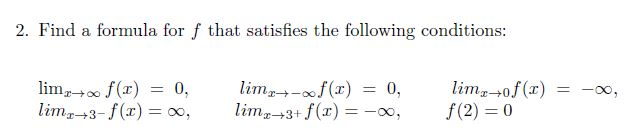help_outlineImage Transcriptionclose2. Find a formula for f that satisfies the following conditions: lim00 f(x) lim-3- f(x) = 0, 0, lim-of(x) = 0, lim3+ f(x) = -0, lim+0f (x) = -00, f(2) = 0 fullscreen
check_circle

Step 1

Rational function

In a rational function we have to find intercepts, asymptotes and end behavior

There are two types of intercepts x-intercept and y-intercept.
At x-intercept, the graph intersects the x-axis similarly at y-intercept, the graph intersects the y-axis.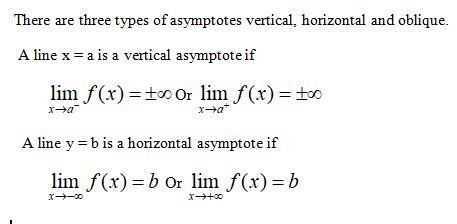Step 2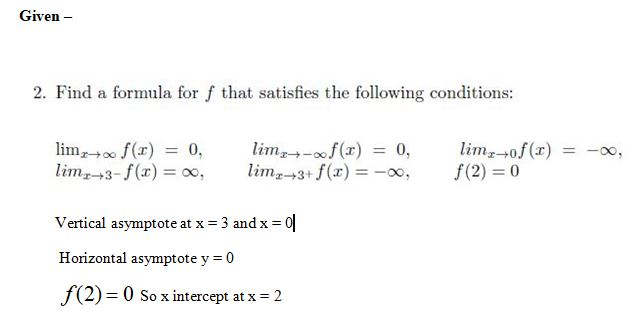Step 3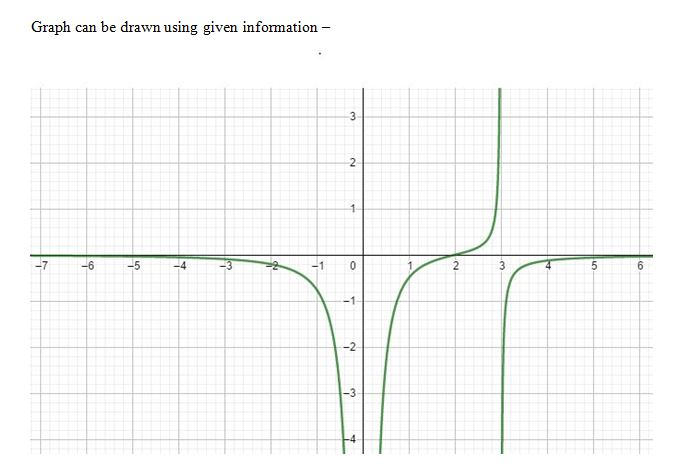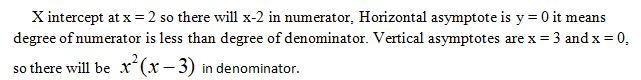...

### Want to see the full answer?

See Solution

#### Want to see this answer and more?

Solutions are written by subject experts who are available 24/7. Questions are typically answered within 1 hour.*

See Solution
*Response times may vary by subject and question.
Tagged in

### Calculus